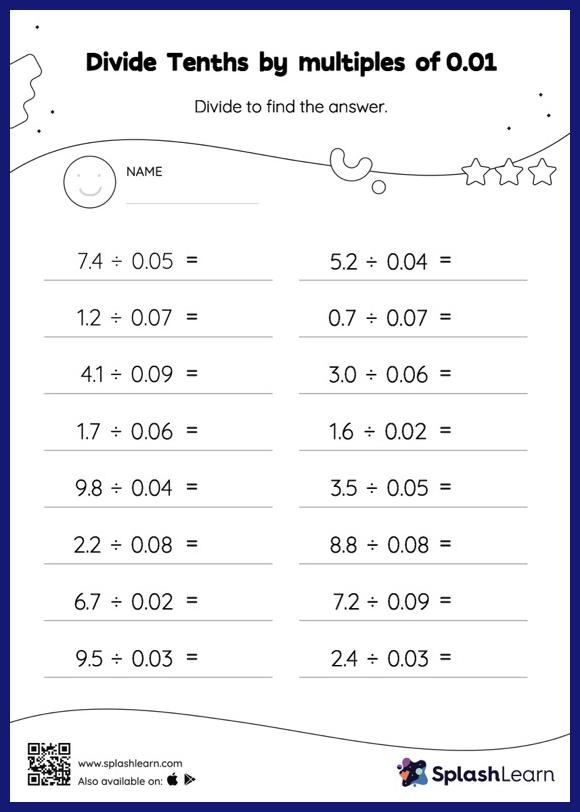# Divide Tenths by multiples of 0.01: Horizontal Division Worksheet

Home > Divide Tenths by multiples of 0.01: Horizontal DivisionWhen students divide two decimals, they do so without considering the decimal point at first. After that, they count the decimal digits in each number and add a decimal point at the appropriate place in the quotient. With the help of this divide tenths by multiples of 0.01 Worksheet, students can practice this idea in great depth. As the worksheet uses a horizontal format, it allows for more creativity when it comes to how the student solves the problem. In contrast, in the vertical format, students usually employ the standard method to solve.